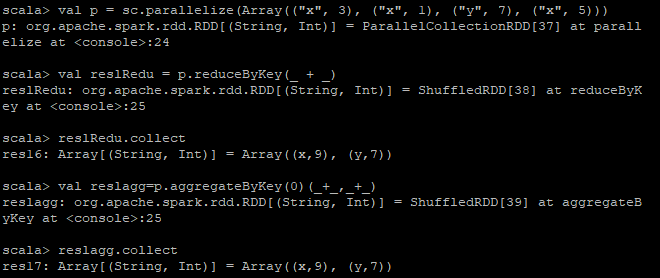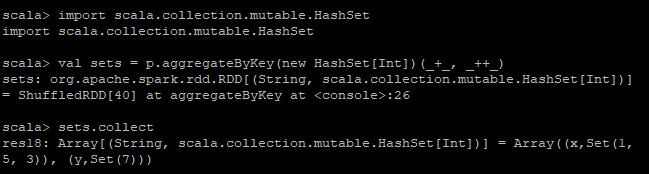2 views

Say I have a distribute system on 3 nodes and my data is distributed among those nodes. for example, I have a test.csv file which exists on all 3 nodes and it contains 2 columns of:

**row   | id,  c.**
---------------
row1  | k1 , c1
row2  | k1 , c2
row3  | k1 , c3
row4  | k2 , c4
row5  | k2 , c5
row6  | k2 , c6
row7  | k3 , c7
row8  | k3 , c8
row9  | k3 , c9
row10 | k4 , c10
row11 | k4 , c11
row12 | k4 , c12

Then I use SparkContext.textFile to read the file out as rdd and so. So far as I understand, each spark worker node will read the a portion out from the file. So right now let's say each node will store:

• node 1: row 1~4
• node 2: row 5~8
• node 3: row 9~12

My question is that let's say I want to do computation on those data, and there is one step that I need to group the key together, so the key value pair would be [k1 [{k1 c1} {k1 c2} {k1 c3}]].. and so on.

There is a function called groupByKey() which is very expensive to use, and aggregateByKey() is recommended to use. So I'm wondering how does groupByKey() and aggregateByKey() works under the hood? Can someone using the example I provided above to explain please? After shuffling where does the rows reside on each node?

by (32.3k points)
edited

groupByKey() is just to group your dataset based on a key. It will result in data shuffling when RDD is not already partitioned.

While aggregateByKey() is logically the same as reduceByKey() but it lets you return the result in different types. In other word, it lets you have input as type x and aggregate result as type y. For example (1,3),(1,2) as input and (1,"six") as output. It also takes zero-value that will be applied at the beginning of each key.

 groupByKey aggregateByKey Does not use Combiner Uses Combiner No parameters as functions. Generally followed by map or flatMap It requires 3 parameters No Combiner Explicit Combiner

AggregateByKey is same as combineByKey and there is a slight difference in functioning and arguments

Here we can pass the initial value, which will be used in each partition

The aggregateByKey function requires 3 parameters:

1. An initial ‘zero’ value will not affect the total values to be collected. For example, if we were adding numbers the initial value would be 0. Or in the case of collecting unique elements per key, the initial value would be an empty set.

• A combining function accepting two parameters. The second parameter is merged into the first parameter. This function combines/merges values within a partition.

• A merging function accepting two parameters. In this case, the parameters are merged into one. This step merges values across partitions.

Example:

Imagine you have a list of pairs. You parallelize it and then you want to "combine" them by key producing a sum. In this case, reduceByKey and aggregateByKey work similarly, as shown below:Now, imagine that you want the aggregation to be a set of values that is of different typesIf you want to know more about Spark, then do check out this awesome video tutorial: